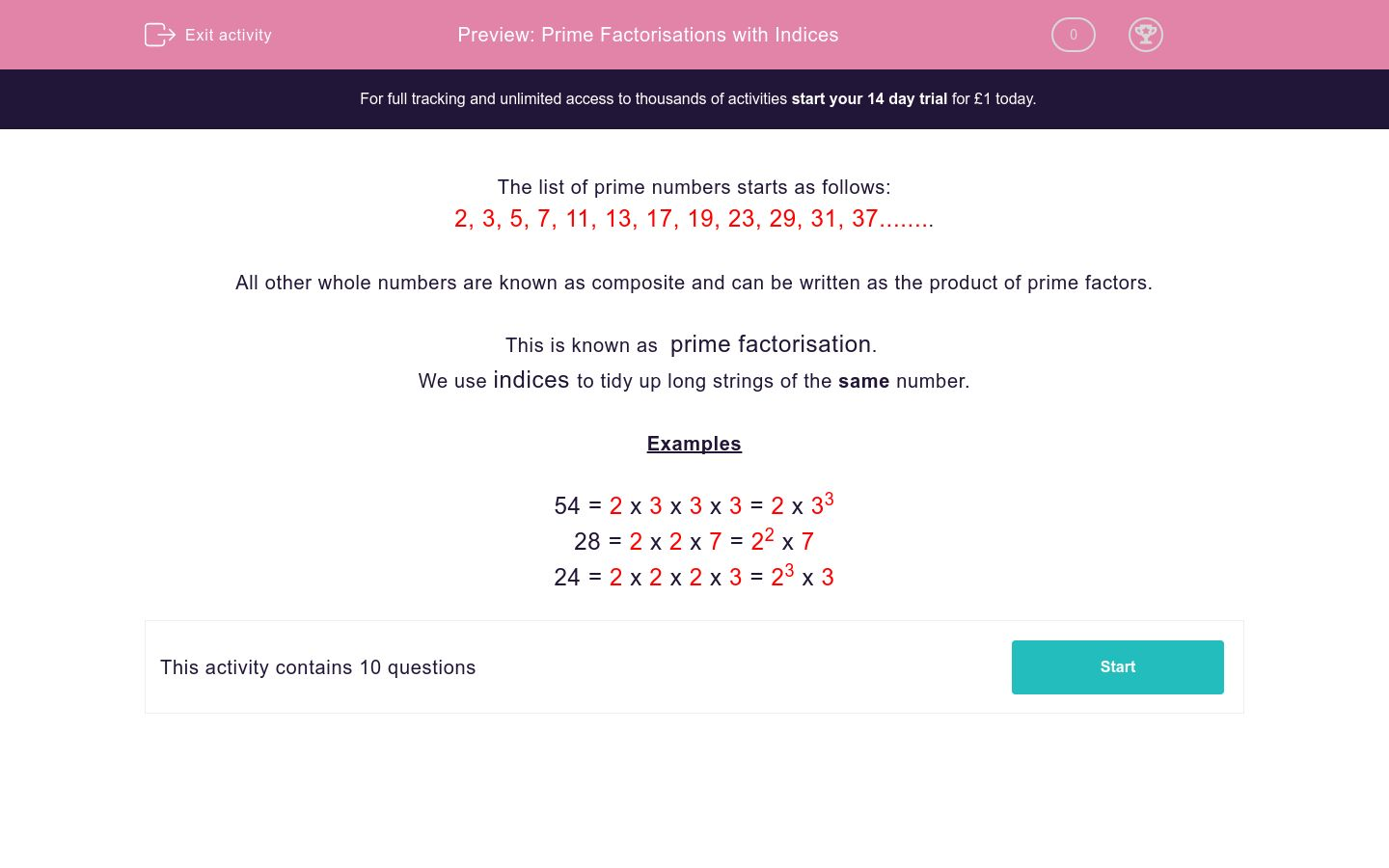# Prime Factorisations with Indices

In this worksheet, students select the correct prime factorisation for the given numbers.Key stage:  KS 2

Curriculum topic:   Maths and Numerical Reasoning

Curriculum subtopic:   Special Numbers

Difficulty level:### QUESTION 1 of 10

The list of prime numbers starts as follows:

2, 3, 5, 7, 11, 13, 17, 19, 23, 29, 31, 37........

All other whole numbers are known as composite and can be written as the product of prime factors.

This is known as  prime factorisation

We use indices to tidy up long strings of the same number.

Examples

54 = 2 x 3 x 3 x 3 = 2 x 33

28 = 2 x 2 x 7 = 22 x 7

24 = 2 x 2 x 2 x 3 = 23 x 3

---- OR ----